# Python | Pandas Index.intersection()

Python is a great language for doing data analysis, primarily because of the fantastic ecosystem of data-centric python packages. Pandas is one of those packages and makes importing and analyzing data much easier.

Pandas` Index.intersection()` function form the intersection of two Index objects. This returns a new Index with elements common to the index and other, preserving the order of the calling index.

Syntax: Index.intersection(other)

Parameters :
other : Index or array-like

Returns : intersection : Index

Example #1: Use `Index.intersection()` function to find the set intersection of two Indexes.

 `# importing pandas as pd ` `import` `pandas as pd ` ` `  `# Creating the first Index ` `idx1 ``=` `pd.Index([``'Labrador'``, ``'Beagle'``, ``'Mastiff'``,  ` `                     ``'Lhasa'``, ``'Husky'``, ``'Beagle'``]) ` ` `  `# Creating the second Index ` `idx2 ``=` `pd.Index([``'Labrador'``, ``'Great_Dane'``, ``'Pug'``, ` `           ``'German_sepherd'``, ``'Husky'``, ``'Pitbull'``]) ` ` `  `# Print the first and second Index ` `print``(idx1, ``'\n'``, idx2) `

Output :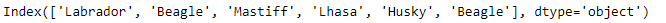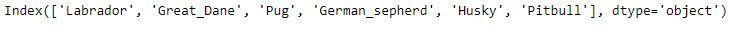Now we find the set intersection of the two Indexes.

 `# Find the elements common to both the Indexes ` `idx2.intersection(idx1) `

Output :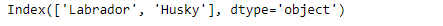As we can see in the output, the `Index.intersection()` function has returned the intersection of the two indexes. The ordering of the labels has been maintained based on the calling Index.

Example #2: Use `Index.intersection()` function to find the set intersection of two Indexes. The Index contains `NaN` values.

 `# importing pandas as pd ` `import` `pandas as pd ` ` `  `# Creating the first Index ` `idx1 ``=` `pd.Index([``'2015-10-31'``, ``'2015-12-02'``, ``None``, ``'2016-01-03'``,  ` `                      ``'2016-02-08'``, ``'2017-05-05'``, ``'2014-02-11'``]) ` ` `  `# Creating the second Index ` `idx2 ``=` `pd.Index([``'2015-10-31'``, ``'2015-10-02'``, ``'2018-01-03'``, ` `           ``'2016-02-08'``, ``'2017-06-05'``, ``'2014-07-11'``, ``None``]) ` ` `  `# Print the first and second Index ` `print``(idx1, ``'\n'``, idx2) `

Output :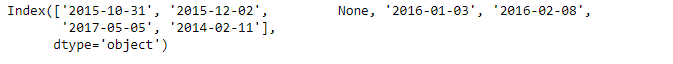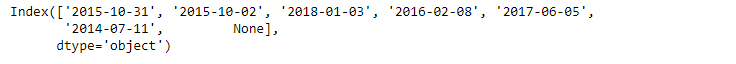Now we find the intersection of idx1 and idx2.

 `# find intersection and maintain  ` `# ordering of labels based on idx1 ` `idx1.intersection(idx2) `

Output :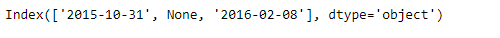Note : The missing values in both the indexes are considered common to each other.

My Personal Notes arrow_drop_upCheck out this Author's contributed articles.

If you like GeeksforGeeks and would like to contribute, you can also write an article using contribute.geeksforgeeks.org or mail your article to contribute@geeksforgeeks.org. See your article appearing on the GeeksforGeeks main page and help other Geeks.

Please Improve this article if you find anything incorrect by clicking on the "Improve Article" button below.

Article Tags :

Be the First to upvote.

Please write to us at contribute@geeksforgeeks.org to report any issue with the above content.